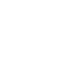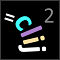# CLIJ2GPU accelerated image processing for everyone

CLIJ2 home

## getSorensenDiceCoefficientDetermines the overlap of two binary images using the Sorensen-Dice coefficent.

A value of 0 suggests no overlap, 1 means perfect overlap. The Sorensen-Dice coefficient is saved in the colum ‘Sorensen_Dice_coefficient’. Note that the Sorensen-Dice coefficient s can be calculated from the Jaccard index j using this formula:

`s = f(j) = 2 j / (j + 1)`

Categories: Measurements, Binary

Availability: Available in Fiji by activating the update sites clij and clij2. This function is part of clij2_-2.5.0.1.jar.

### Usage in ImageJ macro

``````Ext.CLIJ2_getSorensenDiceCoefficient(Image source1, Image source2, Number sorensen_dice_coefficient);
``````

Imprint